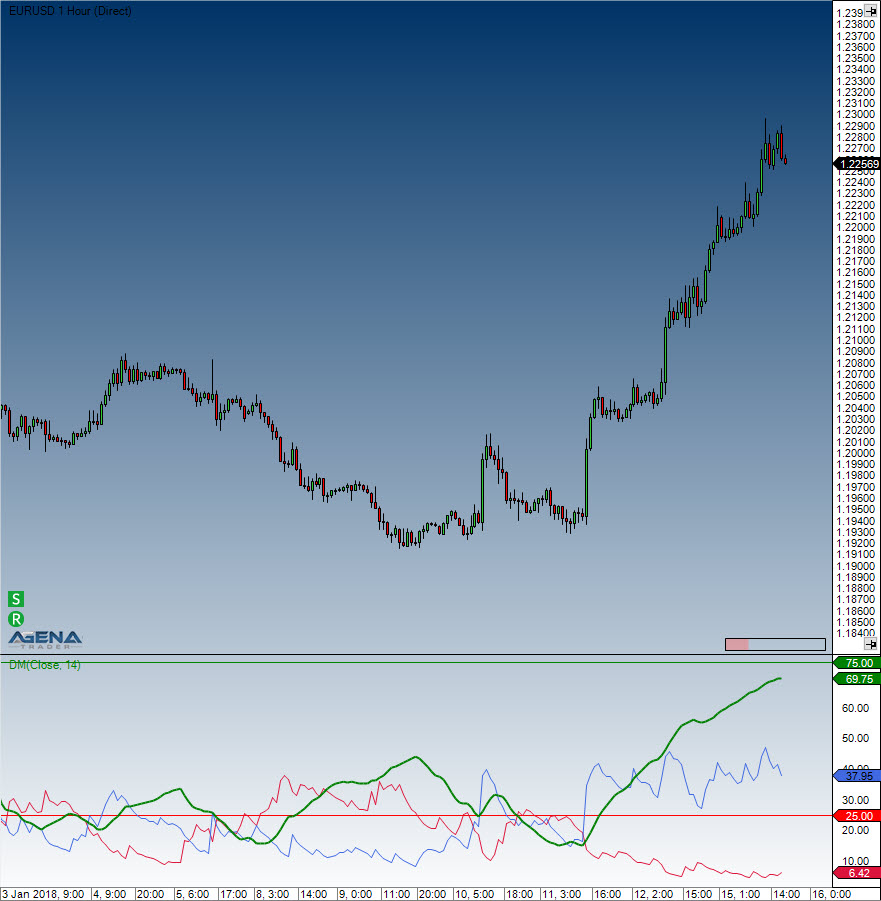Comment on page

# Directional Movement (DM)

#### Description

The Directional Movement indicator is almost identical to the ADX, with the only difference that the +DM and -DM values are also calculated. These values are then later on used for the DMI.

#### Interpretation

The Directional Movement indicator is positive when the difference between the highs is at its largest.

#### Further information

See: Directional Movement Index (DMI)

#### Usage

DM(int period)
DM(IDataSeries inSeries, int period)
DM(int period)[int barsAgo]
DM(IDataSeries inSeries, int period)[int barsAgo]
//For the value of +DM
DM(int period).DiPlus[int barsAgo]
DM(IDataSeries inSeries, int period).DiPlus[int barsAgo]
//For the value of -DM
DM(int period).DiMinus[int barsAgo]
DM(IDataSeries inSeries, int period).DiMinus[int barsAgo]

#### Return value

double
When using this method with an index (e.g. DM(14).DiPlus[int barsAgo] ), the value of the indicator will be issued for the referenced bar.

#### Parameters

inSeries Input data series for the indicator
period Number of bars included in the calculations

#### VisualizationDirectional Movement (DM)

#### Example

//Output of the DM values
Print("The current +DM value is: " + DM(14).DiPlus);
Print("The current –DM value is: " + DM(14).DiMinus);